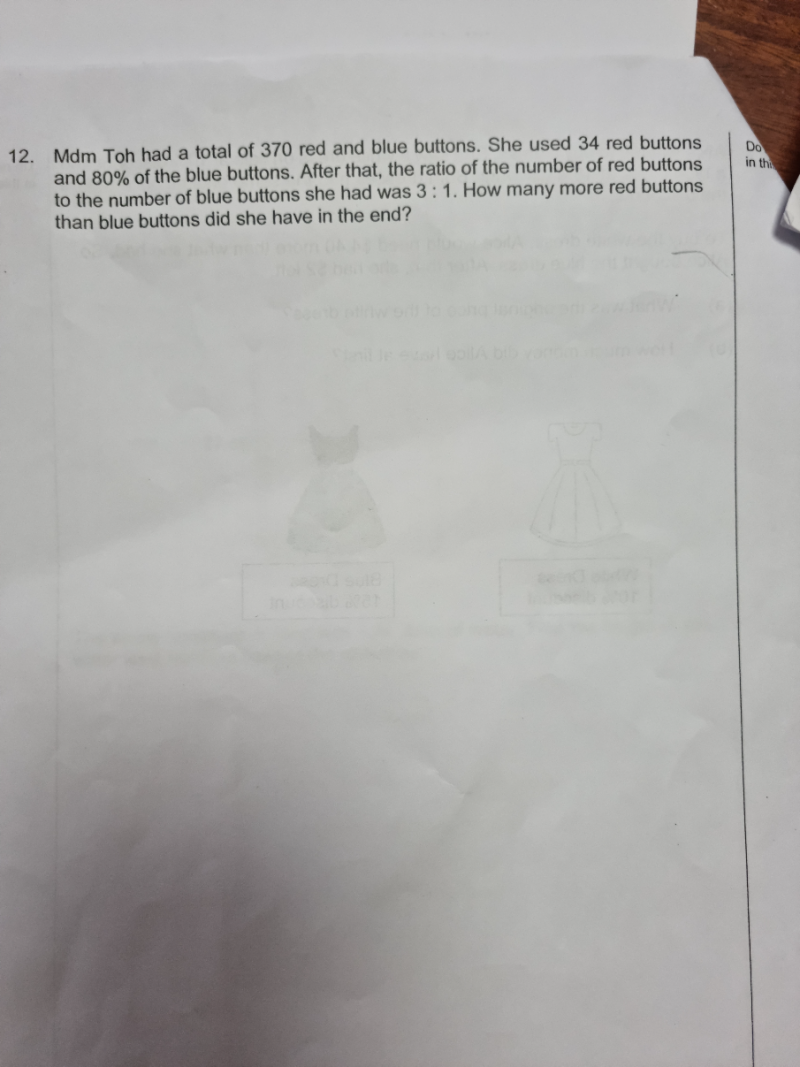# QuestionThank you

Red buttons                  Blue buttons              Total

At first              370-u                                      u                          370

Used                    -34                                      0.8u

End ratio              3                                            1

Red buttons left = 370-b-34 = 336-u

Blue buttons left = 0.2u

(336-u) / 0.2u    =  3  ( or ratio 3/1)

336-u = 0.6u

1.6u = 336 => u = 210

Red buttons in the end = 370-u-34 = 370-210-34 = 126

Blue buttons in the end = 0.2(210) = 42

Hence red buttons more than blue buttons in the end = 126-42 = 84